Search
 Submission Procedure share: |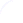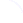Follow usavailable in:   PDF (32 kB) PS (194 kB)

get:
 Similar Docs BibTeX Write a comment
get:
 Links into Future

DOI:   10.3217/jucs-002-02-0087

# On the Scalability of Molecular Computational Solutions to NP Problems

Dónall A. Mac Dónaill,
(Department of Chemistry & Centre for Scientific Computation,
Trinity College, University of Dublin, Ireland
dmcdonll@tcd.ie)

Abstract: A molecular computational procedure in which manipulation of DNA strands may be harnessed to solve a classical problem in NP - the directed Hamiltonian path problem - was recently proposed [Adleman 1994], [Gifford 1994]. The procedure is in effect a massively parallel chemical analog computer and has a computational capacity corresponding to approximately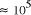CPU years on a typical 10 MFLOP workstation. In this paper limitations on the potential scalability of molecular computation are considered. A simple analysis of the time complexity function shows that the potential of molecular systems to increase the size of generally solvable problems in NP is fundamentally limited to. Over the chemically measurable picomolar to molar concentration range the greatest practical increase in problem size is limited to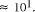Key Words: Molecular Computation, DNA, NP-problem

# 1 Introduction

The (directed) Hamiltonian Path Problem (HPP), first proposed by William Rowan Hamilton, is one of the most troublesome problems in Graph theory. Very similar to the Euler path problem, it may be stated as follows: for a graph G with vertices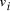, and edges, is there a path which starting and ending at specified vertices, visits each remaining vertex once and only once?

A trial and error approach is theoretically possible but proves impossible in practice for all but the smallest graphs; the number of paths which it is necessary to check simply grows too rapidly. In fact the HPP problem belongs to a class of problems called NP-complete, which rapidly become too complex to be solved by standard (deterministic) computers.

Problems of this nature are of considerable importance in computer science. There was therefore considerable interest in a recent report by Adleman which suggested that modern laboratory techniques of molecular biology could be employed to manipulate strands of DNA so as to solve the HPP [Adleman 1994]. A subsequent study has suggested how the method may be adapted for the solution of another classical problem in NP - the "satisfiability" or SAT problem [Lipton 1995].

Page 87

Adleman's insight lay in exploiting the parallelism inherent in chemical systems to yield a massively parallel analog computer, in a brute force approach to solving complex problems in NP. However, while it was noted that chemical systems - and thus molecular computers - can be easily scaled in size, the fundamental question of the scalability of problems solvable by molecular computation was left open. This paper addresses this central issue and, because the discussion draws on the diverse disciplines of molecular biology and computer science, begins with a brief review of both problem classification and Adleman's experiment.

## 1.1 Classification of Problem Complexity

Time complexity functions describe how the cost of solving a problem grows on increasing the size (or input length), n, of the problem. Computer scientists recognize two distinct classes [Garey and Johnson 1979]. Algorithms for which the time complexity function is O(p(n)), where p(n) is a polynomial in n, are termed polynomial time algorithms, whereas algorithms with no polynomial bound on their complexity are called exponential time algorithms. There is a remarkable difference in growth rates between polynomial and exponential algorithms [Fig. 1].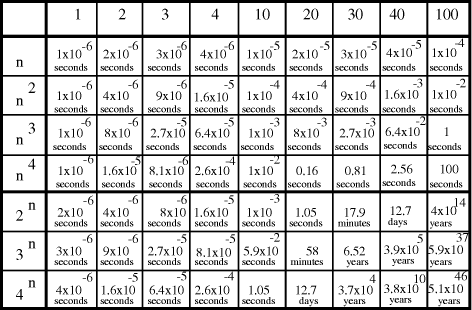Figure 1: Time complexity functions for some polynomial (n,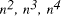) and exponential (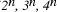) functions as a function of problem size, n.

Page 88

Problems with polynomial algorithms are considered "good", whereas problems which are so difficult that no polynomial time algorithm can solve them are termed "intractable".

The theory of NP-completeness is concerned with decision problems. Informally, a decision problem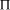is said to belong to the class P if there exists a Deterministic Turing Machine (DTM) program which solves the problem in polynomial time. Non-deterministic computation differs from deterministic computation in that a solution is exhaustively guessed and then checked. If a correct solution to a decision problem can be checked in polynomial time, then that problem is said to belong to the class NP (Non-deterministic Polynomial time). Since problems which can be solved deterministically in polynomial time can also be guessed non-deterministically and checked in polynomial time, we can write P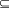NP [Fig. 2]. It is not known whether this inclusion is proper, that is whether NP\P is occupied.

A practical difficulty with problems in NP is that their time-complexity with a deterministic algorithm is exponential. Thus problems in NP are intractable with deterministic machines.

Certain problems in NP have the property that all other problems in NP may be polynomially reduced to them, and are termed NP-complete. If a polynomial time algorithm can be found for any member of this equivalence class then one can be found for all problems in NP. In other words, if for any (problem)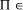NP-complete, then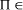P if and only if P = NP. ProblemsNP-complete may be regarded as the hardest problems in NP. The relationship between P, NP and NP-complete is illustrated below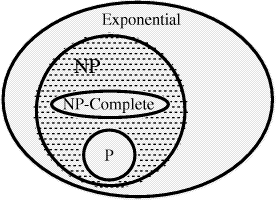Figure 2: Classification of problem complexity

Not surprisingly the class of NP-complete problems has been the focus of considerable attention; including some recent ideas on "Quantum Computers" [DiVincenzo 1995] which could in principle solve NP problems in polynomial time. Several classical problems such as the Hamiltonian Path problem, the satisfiability problem have been shown to be NP-complete. For a more complete

Page 89

discussion of these points the reader is referred to the work of Garey and Johnson [Garey and Johnson 1979].

## 1.2 Molecular Computation and the HPP

The following is a simple non-deterministic algorithm for solving the HPP [Adleman 1994]:

• (Step I) generate random paths in the graph
• (Step II) keep only those paths with begin and end at the specified vertices.
• (Step III) for an n vertex graph keep only those paths with n vertices.
• (Step IV) keep only those paths which visit each vertex once.
• (Step V) if any paths remain then those paths are Hamiltonian and the answer to the HPP is "Yes". Otherwise "No".

Adleman demonstrated, using a graph small enough to be solved by visual inspection [Fig. 3], that DNA could be used to solve the Hamiltonian Path Problem (NP-complete).Figure 3: The directed graph used by Adleman. For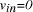and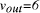the edges 0-1, 1-2, 2-3, 3-4, 4-5, 5-6, define a Hamiltonian path.

20-mer oligonucleotides were associated with each vertex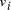in the graph. Oligonucleotides representing edges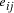, linking vertices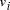and, consisted of the 3' 10-mer of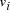and the 5' 10-mer of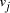[Fig. 4]. The highly specific binding of nucleotides A with T and C with G are such that strands which are Watson-Crick complementary to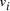, denoted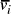, serve to link compatible edges [Fig. 4]. Thus, ligation reactions can only generate nucleotide polymers corresponding to random paths on the graph.

Page 90

Step I is the most critical step in the algorithm as it is essential that all paths be explored, a task with exponential time complexity on a deterministic machine. Adleman addressed this by employing 50 picomole quantities of nucleotides corresponding to edges and the Watson-Crick complement of vertices. While 50 picomole quantities are minute in chemical terms, the parallelism of chemical systems is such that they correspond tocopies of each edge and vertex - presumed sufficient to ensure that polymers corresponding to all paths in the graph are generated.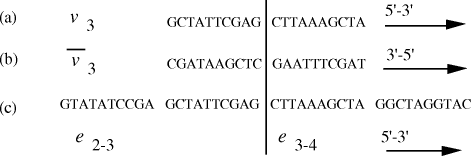Figure 4: (a) DNA strand corresponding to vertex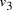. (b) Watson-Crick complement of vertex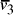. (c) strands corresponding to edgesand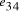. Nucleotide specificity in binding (A-T and C-G) ensures that vertexserves to bind edges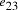and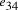.

The remaining steps (II-V) in the algorithm were executed using standard laboratory techniques. Polymers corresponding to paths between the specified starting vertex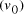and finishing vertex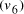were selected (Step II) and amplified (i.e. concentration increased) by using the polymerase chain reaction (PCR). Argose gel electrophoresis was used to identify those polymers corresponding to paths involving n (= 7) vertices (Step III), and the product was again amplified using the PCR. Step IV was implemented using affinity purification for oligonucleotide sequences corresponding to vertices 1, 2, 3, 4, 5 and 6. Any remaining polymer must correspond to a Hamiltonian path, and a suitably primed PCR was employed to detect and amplify any such polymer.

# 2. Scalability of Molecular Computation

## 2.1 Computational Capacity of Molecular Computers

In Adleman's original paper the question of the scalability of problems solvable by molecular computation was left open [Adleman 1994]. The system contained 14 edges, each represented by 50 picomoles of nucleotide, corresponding to a total of 5 x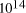edge nucleotides. Associating each nucleotide binding with a computation, Adleman's molecular system yielded a total ofPage 91

computations. Concentrations could easily be scaled to yield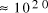computations [Adleman 1994], which may be easily shown to correspond toCPU years on a typical 10 MFLOP workstation.

Given this phenomenal computational capacity, and the ease with which chemical systems can be scaled, it would be easy for the non-specialist to conclude that the potential for molecular computation is practically unlimited. This is not the case; [Lineal and Lineal 1995], [Bunow 1995] and [Lipton 1995] have identified problems in the potential scalability of Adleman's method. The following analysis quantifies these limitations.

Problems in NP, among them the directed Hamiltonian Path problem, have a time complexity of, where n is the ''size`` of the problem and p(n) a suitably defined polynomial in n [Garey and Johnson 1979]. Taking the best case situation, p(n) = n, the cost of solving a problem of size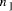using a given computational resource is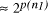. Increasing the scale of the molecular system by a factor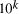allows for the solution of larger problems, the size of which,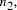, is given by solving the equalityand the increase in the size of solvable problem,, is given by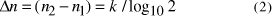## 2.2 Size of Molecular Computers

The limitations expressed in equation (2) on the capacity of molecular computers to solve problems in NP are forcefully illustrated by considering limits on the scale of chemical systems. In practice, electroanalytical techniques allow for the detection of chemicals in the picomolar range [Dong and Wang 1988]. However, if we set aside this technological limit, and assume that individual molecules can in principle be detected (as in scanning tunnelling microscopy), then the smallest possible molecular computers would consist of order unity molecules.

The largest conceivable molecular computer would involve all particles in the universe. Of course, the amount of matter in the universe is not known, but it may be reasonably estimated as follows: Taking Hubbel's constant as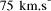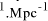[Kaufmann 1994] the age of the universe is 1.3 xyears or 4.1 x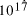seconds. Expanding at the speed of light this gives the volume of the universe as 7.9 x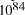. Further assuming a universe with critical density,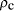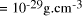[Kaufmann 1994], i.e. a universe which has just sufficient matter to be closed, the mass of the universe may be estimated as 8.7 x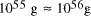. This is the equivalent of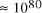particles of mass 1 a.m.u. (taking Avogadro's number as 6.02 x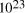).

Page 92

Assuming therefore for the purposes of illustration that the universe contains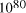particles, it follows that the size of molecular systems can span a maximum 80 decades, and the greatest possible increase in solvable problem size is given by equation (2) as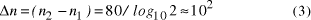Thus the potential to increase the size of problem (in NP) which may be solved, by increasing the size of the chemical system, is very modest indeed, even when fantastic scales are involved.

More relevantly, over the realizable/detectable picomolar to molar concentration range we haveThus while the size of molecular computers can be readily increased, the increase in the size of problems which such systems could solve is much more modest. The scalability of problem size with the size of molecular system employed is illustrated in Fig. 5.

Page 93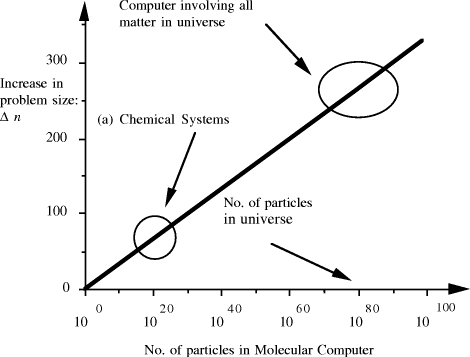Figure 5: Increase in solvable problem size with increase in the size of the molecular computer (relative to the size of problem solvable with a system of order unity molecules).

# 3 Conclusion

The potential of molecular systems to increase the size of solvable problems in NP is fundamentally limited to the order of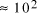. Nevertheless, the computational capacity of Adleman's approach is enormous, equivalent toCPU years on a 10 MFLOP workstation. The analysis is general and based on the time complexity function, which gives the time in which a solution is guaranteed to be found, and is therefore a worst possible case analysis; faster solutions may be available in many cases. It is not therefore suggested that there will be no instances where molecular computers will prove superior to electronic computers.

# References

[Adleman 1994] Adleman, L.: "Molecular Computation of Solutions to Combinatorial Problems"; Science, 266, (1994), 1021-1024.

[Bunow 1995] Bunow, B.: "On the Potential of Molecular Computing"; Science, 268, (1995), 481-482.

[DiVincenzo 1995] DiVincenzo, D. P.: "Two-Bit Gates are Universal for Quantum Computation", Phys. Rev. A, 51, (1995), 1015-1022.

[Dong and Wang 1988] Dong, S., Wang, Y.: "A Nafion/Crown Ether Film Electrode and its Application in the Anoidic Stripping Voltametric Determination of Traces of Silver"; Anal. Chim. Acta, 212, (1988), 341-347.

[Garey and Johnson 1979] Garey, M. R., Johnson, D. S.: "Computers and Intractability"; Freeman, New York, (1979).

[Gifford 1994] Gifford, D. K.: On the Path to Computation with DNA, Science, 266, 1994, 993-994.

[Lineal and Lineal 1995] Lineal, M., Lineal, N.: "On the Potential of Molecular Computing", Science, 268, (1995), 481.

[Lipton 1995] Lipton, R. J.: "DNA solution of Hard Combinatorial Problems", Science, 268, (1995), 542-545.

[Kaufmann 1994] Kaufmann, W. J.: "Universe"; Freeman, New York, (1994).

Acknowledgments

The author would like to thank Dr. T. Tuohy (Department of Molecular Genetics, Biochemistry and Microbiology, University of Cincinnati, Ohio), Dr. J. Greer

Page 94

(Department of Chemistry, Trinity College Dublin) and Dr. H. Gibbons (Department of Computer Science, Trinity College Dublin), for useful discussions.

Page 95